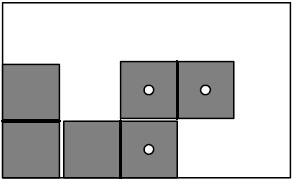Online JudgeProblem SetAuthorsOnline ContestsUser
Web Board
F.A.Qs
Statistical Charts
Problems
Submit Problem
Online Status
Prob.ID:
Register
Authors ranklist
Current Contest
Past Contests
Scheduled Contests
Award Contest
Register
Language:
Packing Dominoes
 Time Limit: 1000MS Memory Limit: 65536K Total Submissions: 291 Accepted: 51 Special Judge

Description

A domino is a 2 x 1 oblong comprising two squares, each square marked with a set of "pips" denoting a non-negative integer. A blank square denotes the number 0 (zero).

In a standard " double-K " set of dominoes, each pair of integers in the range 0...K occurs on one domino. In other words, the dominoes are numbered:

(0, 0),(0, 1),...(0, K),(1, 1),...(1, K),(2, 2),...,(K, K)

Given a set of dominoes and a rectangular area of width w and height h , write a program to pack that entire set of dominoes into that area subject to the limitation that, whenever two dominoes touch one another either horizontally or vertically, the numbers on the touching squares must be identical. Note that the area might not be completely covered, and, space permitting, some dominoes might not touch any others.

Input

Input will consist of one or more lines, each containing 3 positive integers. The first integer, K , indicates the size of the set of dominoes - a "double-K set" as described above. The remaining integers, w and h , indicate the size of the area in which the dominoes should be placed, measured in units the same size as one of the constituent squares of a domino. The end of input will be signaled by a non-positive K value.

Output

For each line of input, print one line containing the values K(K<=3) , w , and h (w,h<=12), as defined above, followed either by a line with the string ``No packing is possible" or by a report consisting of (K+1)(K+2)/2 lines, each line describing one domino, ordered as described above in the definition of a ``doubleK " set.

Each line of that report will contain 6 integers

d1 d2 x1 y1 x2 y2

where d1 and d2 are the numbers on that domino, (x1, y1) is the coordinates of the number d1 in the packed layout, and (x2, y2) is the coordinates of number d2 . Coordinates are specified as integers with (0,0) denoting the lower-left corner of the packing area.

If multiple packings are possible, any packing meeting the criteria outlined above will be accepted.

Sample Input

```1 5 3
3 5 2
-1 0 0```

Sample Output

```1 5 3
0 0 0 0 0 1
0 1 1 0 2 0
1 1 3 0 3 1
3 5 2
No packing is possible

```

Hint

Note: This output corresponds to the packing shown here and is only one of many possible correct responses.Source

[Submit]   [Go Back]   [Status]   [Discuss]Home PageGo BackTo top